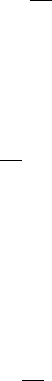6Fourier transforms

IB Methods6.6 Linear systems and response functions
Suppose we have an amplifier that modifies an input signal
I
(
t
) to produce an
output
O
(
t
). Typically, amplifiers work by modifying the amplitudes and phases
of specific frequencies in the output. By Fourier’s inversion theorem, we know
I(t) =
1
2π
Z
e
t
˜
I(ω) dω.
This
˜
I(ω) is the resolution of I(t).
We specify what the amplifier does by the transfer function
˜
R
(
ω
) such that
the output is given by
O(t) =
1
2π
Z
−∞
e
t
˜
R(ω)
˜
I(ω) dω.
Since this
˜
R
(
ω
)
˜
I
(
ω
) is a product, on computing
O
(
t
) =
F
1
[
˜
R
(
ω
)
˜
I
(
ω
)], we
obtain a convolution
O(t) =
Z
−∞
R(t u)I(u) du,
where
R(t) =
1
2π
Z
−∞
e
t
˜
R(ω) dω
is the response function. By plugging it directly into the equation above, we see
that R(t) is the response to an input signal I(t) = δ(t).
Now note that causality implies that the amplifier cannot “respond” before
any input has been given. So we must have R(t) = 0 for all t < 0.
Assume that we only start providing input at t = 0. Then
O(t) =
Z
−∞
R(t u)I(u) du =
Z
t
0
R(t u)I(u) du.
This is exactly the same form of solution as we found for initial value problems
with the response function R(t) playing the role of the Green’s function.